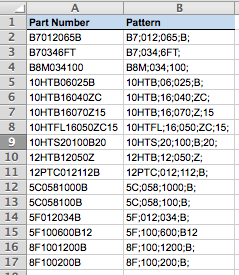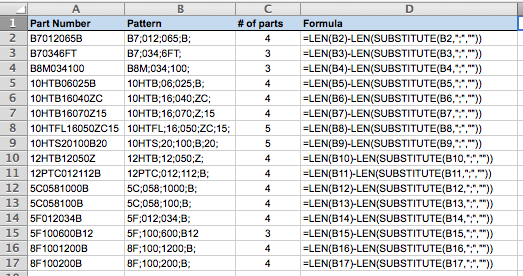# Count the occurrences of a single character in a cell in Excel

This lesson shows you a way to calculate the number of times a single character occurs in a cell in Excel, and provides a real-life example where I needed to split a column of cells containing part numbers into individual components for each part number.

In this example, I was given a spreadsheet with a number of part number patterns. These patterns were off different lengths, and were separated into a number of individual components, each separated by a semi-colon. Part of my job involved figuring out how many components each pattern contained. Here's an example from the spreadsheet I was given:As you can see from the example above, each part number is different. In order to split each into its component parts, the first thing I need to do is write a formula to determine how many parts each pattern has.

To do this, I used the following formula:

`=LEN(B2)-LEN(SUBSTITUTE(B2,&quot;;&quot;,&quot;&quot;))`

Here's how my spreadsheet looked once I'd done this:Let's break down the formula to see how it works:

 `=LEN(B2)` This element of the formula counts the number of characters in B2 `SUBSTITUTE(B2,";","")` This element of the formula uses the SUBSTITUTE() function to replace all of the semi-colons in B2 with nothing (the third element in this formula, "", means "nothing". The result is a text value that doesn't have any semi-colons in it. We can then use the LEN() function again to find out how long the resulting text string is (see below). `LEN(SUBSTITUTE(B2,";",""))` By putting the LEN() function around the SUBSTITUTE() function, Excel calculates the length of the text string that is left behind if all the semi-colons are removed. By subtracting this from the first element, which was the length of the original text value, we can work out how many semi-colons were removed.

This may seem more complicated than it needed to be. For a start, I could have simply subtracted LEN(A2) from LEN(B2). However, you won't always have all the data you need (or want) so the formula outlined in this lesson provides a more flexible way to achieve the result you're after.

## Our Comment Policy.

Add a comment to this lesson

## Comments on this lesson

### What an elegant solution -

What an elegant solution - thanks for this.

### Thank you!

Thanks for your great solution! I used it in a slightly different context that might be of interest. I have a sheet where column A is mainly numbers in a Work Breakdown Structure style (1, 1.1, 1.1.1, etc.) and wanted a simple way to differentiate the levels without a lot of manual formatting.

Your single-formula approach works very well as a conditional format. I selected column A and applied Conditional Formatting. I selected Use a Formula to Determine Which Cells to Format and created the following formula:

=LEN(A1)-LEN(SUBSTITUTE(A1,".",""))=0 (there are 0 periods in the current cell, so it's a top-level task) make it bold

I did that a couple more times:

=LEN(A1)-LEN(SUBSTITUTE(A1,".",""))=1 (there is 1 period in the current cell) make it blue
=LEN(A1)-LEN(SUBSTITUTE(A1,".",""))=2 (means there are 2 periods in the current cell) make it red
etc. as necessary

Now when I hand the sheet off to someone, they just type in the number and the rest takes care of itself. I only wish I could indent using Conditional Formatting instead of VBA.

### GREAT

Your solution worked for me... Thank You!